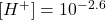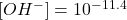## What are the concentrations of hydronium and hydroxide ions in a solution with pH 2.6? Please help omg. Ive been stuck for 15 min

Question

What are the concentrations of hydronium and hydroxide ions in a solution with pH 2.6?

in progress 0
6 months 2021-07-11T20:19:26+00:00 1 Answers 3 views 0

The concentrations of hydronium and hydroxide ions in a solution with pH 2.6 are 2.51*10⁻³ and 3.98*10⁻¹² respectively.

Explanation:

pH is a measure of the acidity or alkalinity of an aqueous solution. The pH indicates the concentration of hydrogen ions present in certain solutions. Mathematically it is defined as the opposite of the base 10 logarithm or the negative logarithm of the activity of hydrogen ions, whose equation is

pH= -log [H⁺]

So, being pH= 2.6 and replacing in the definition of pH:

2.6= -log [H⁺][H⁺]=2.51*10⁻³

pOH is a measure of the concentration of hydroxide ions (OH⁻). The sum between the pOH and the pH results in 14:

pOH + pH= 14

So, being pH= 2.6:

pOH + 2.6= 14

pOH= 14 – 2.6

pOH= 11.4

The pOH is defined as the negative logarithm of the activity of the hydroxide ions. That is, the concentration of OH⁻ ions:

pOH= – log [OH⁻]

Being pOH= 11.4 and replacing

11.4= -log [OH⁻][OH⁻]= 3.98*10⁻¹²

The concentrations of hydronium and hydroxide ions in a solution with pH 2.6 are 2.51*10⁻³ and 3.98*10⁻¹² respectively.